## 6.4.2 Tin Oxide Deposition using PSD Pyrolysis

The deposition of tin oxide (SnO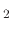) on silicon dioxide using the spray pyrolysis deposition process was performed by one of our industrial partners using an air atomizer which is not located directly above the wafer, but rather on the side, as depicted in Figure 6.18.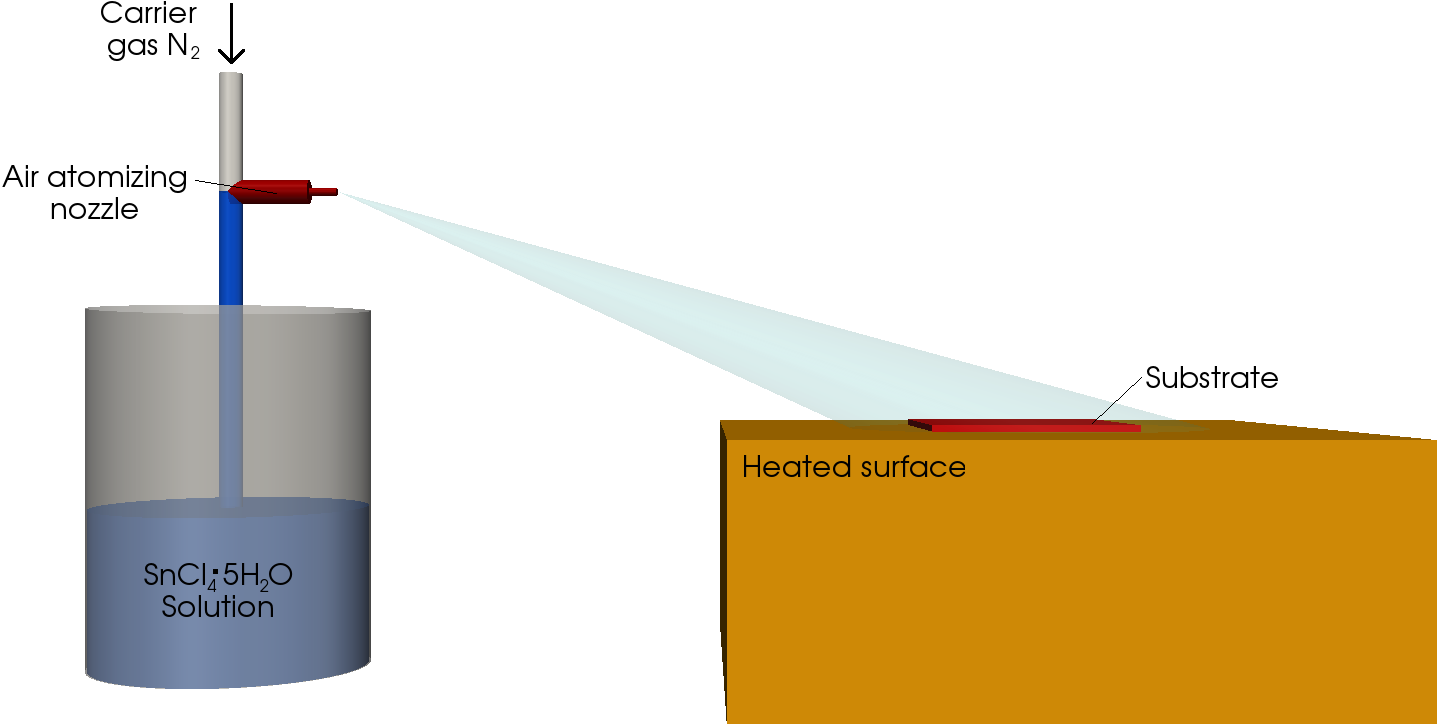The nitrogen pressure of the atomizer was set to 2bar in air and 0.7bar in the liquid. These values are outside of the data sheet for the nozzle used , which is done in order to obtain smaller droplet sizes and slower deposition rates . However, the data sheet information was extracted and the provided graph extended in order to find an approximate radial distribution of particles. The simulated radius of particles is set between 1.5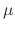m and 5.5m. The spray direction is also mentioned in the data sheet; however, once again, the implemented pressure combination is not found in the available documentation and an extrapolated estimate must be used. The spray nozzle in use is one which produces a flat spray pattern with droplet dispersal proceeding mainly in the lateral axial direction. The vertical direction experiences much less particle dispersal; however, some dispersal does occur and the ratio between the lateral and vertical dispersal is approximated to 10:1. The nozzle is approximately 20cm laterally and 10cm vertically distanced from the substrate and the spray is directed such that much of it is found above the heated surface, where it can deposit onto the wafer. The parameters related to water droplets and the PSD simulation environment can be found in Table 6.1. The initial velocity of the droplets, as they are pushed out of the atomizer is not provided by the manufacturer . Therefore, an approximation is made based on values found in literature which, as previously shown in Section 4.1 suggest an initial particle velocity between 5m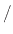s and 20ms.

As an example, a particle with a 5m radius is analyzed. Its initial velocity is assumed to be 20ms, fully in the horizontal direction. Therefore, the only forces which the particle experiences are the gravitational force and the Stokes force during its vertical flight and the Stokes force during its lateral flight towards the wafer sample. The droplet's vertical downward acceleration due to gravity is given by 9.81ms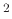. The Stokes force is given by (4.7) and for the sample droplet is valued at 2.07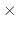10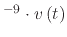N. The mass of the droplet is given by (4.2) and is calculated to 52310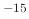kg. Therefore, the negative acceleration due to the stokes force is approximately 3960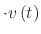ms, which is a very large negative force. When a particle is accelerated with an initial velocity of 20ms, it will instantaneously experience a negative acceleration of 79.210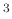ms. This means that it is not possible for the droplet to travel the required distance when starting with the stated initial velocity. In fact, the initial velocity must follow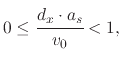(205)

whereis the required distance,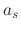is the velocity-dependent factor of the Stokes negative acceleration, and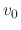is the initial velocity. Therefore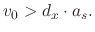(206)

In order for the sample droplet to reach the required distance to the wafer, a minimal initial velocity of 1.1110ms is required. An alternative is the introduction of an additional force, such as is the case, when ESD deposition is performed as described in the previous section. It is evident that the initial velocity suggested in the literature is one which assumes no introduction of an additional air pressure. The additional pressure serves to break up the droplets due to an instantaneous increase in velocity and to introduce an additional push'' which gives the droplets the appropriate initial velocity in order for them to reach a range beyond several millimeters.

It may be noted that after leaving the atomizer, the droplets do not travel in the same manner together as they would individually. The interaction between droplets for the ESD system was ignored since, in literature , , it was suggested that they do not affect the droplet motion significantly. In the ESD system, the electric field is the main factor to the droplet's movement. However, for the pressure system described here, it appears that interaction between droplets plays a larger role. Droplets move through air as a flux and calculating individual droplet's movements in order to find their final location on the wafer surface does not produce a match to the experimental results, shown in Figure 6.19a from .

Figure 6.19: Images showing the deposited SnOfilm as a results of a PSD deposition step. The good step coverage confirms a chemical and not physical reaction takes place during deposition.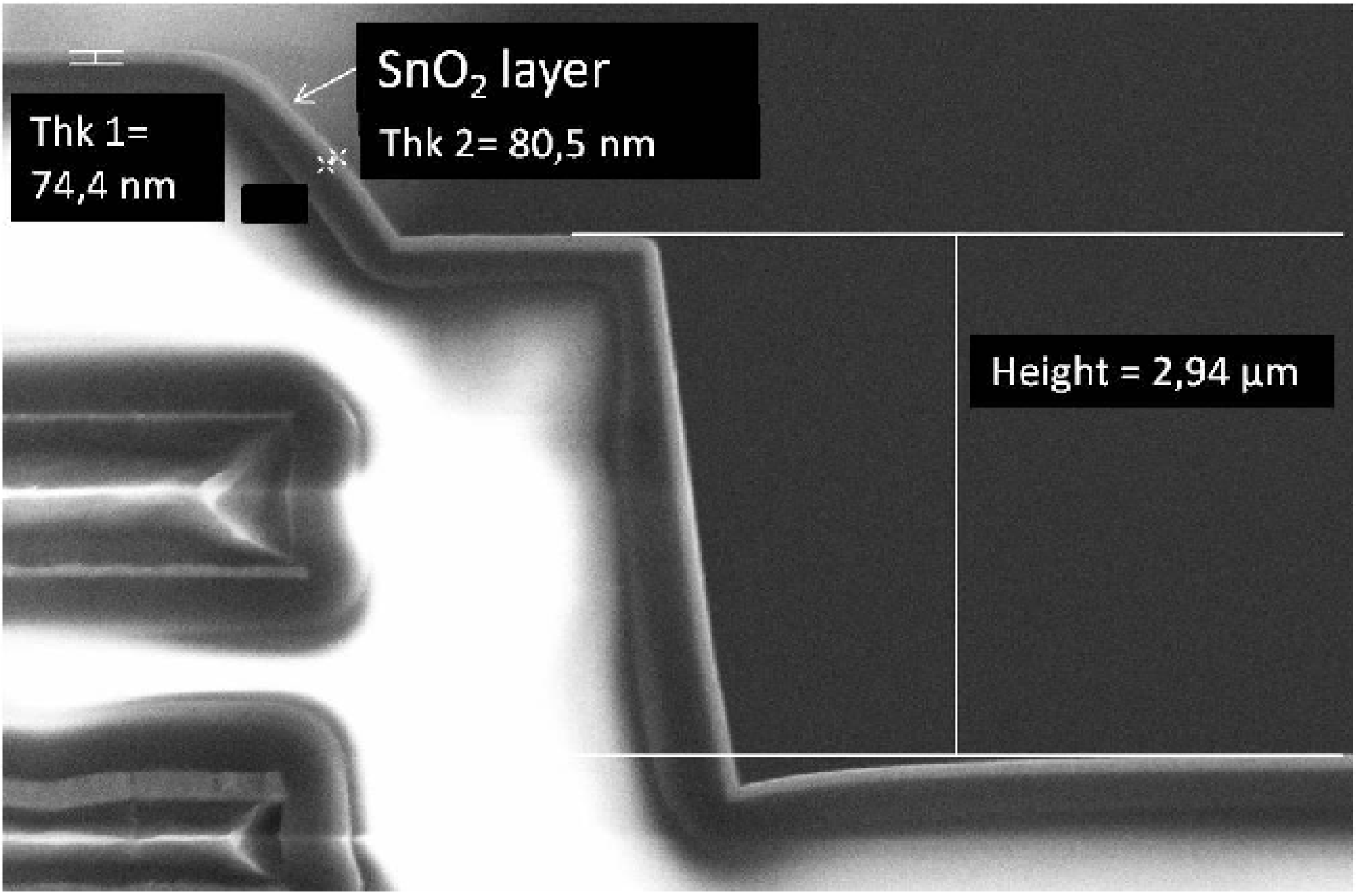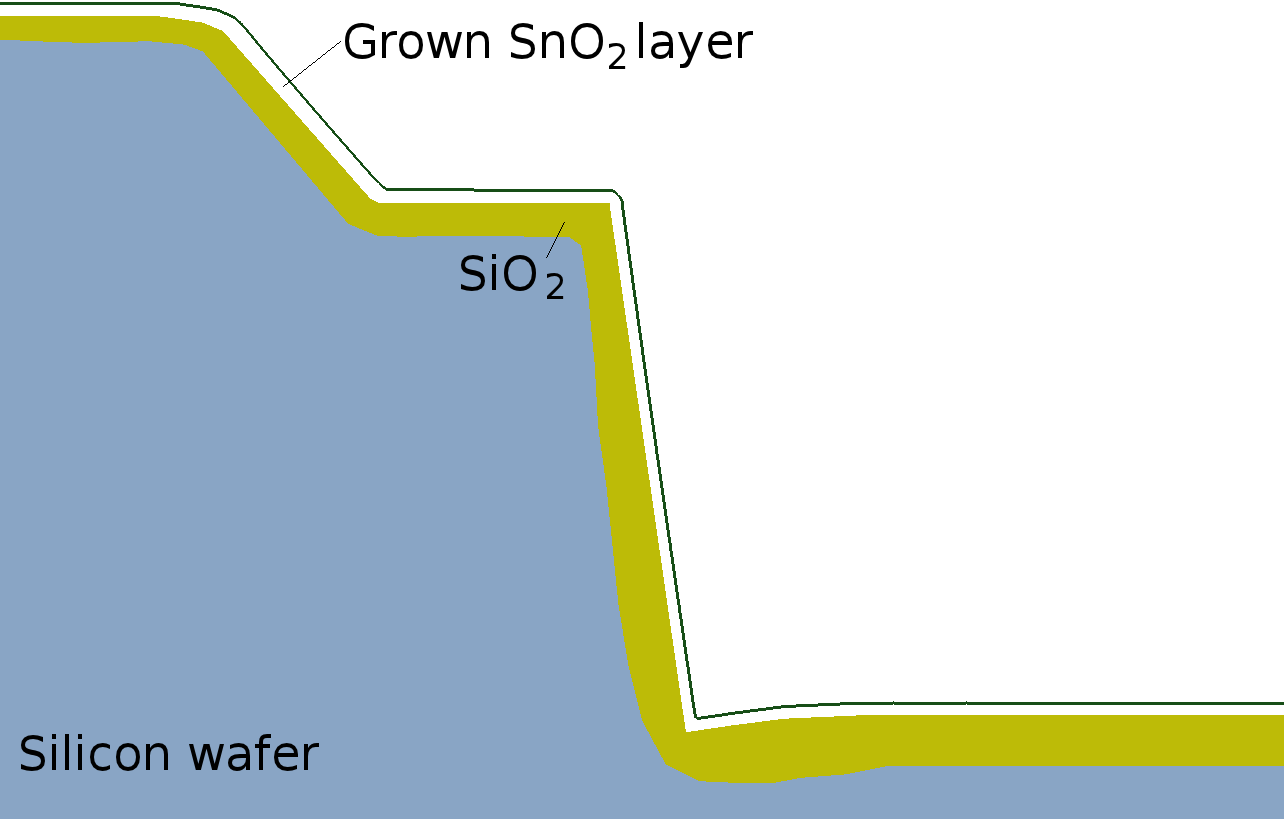(a) SEM image (b) Simulation

There are also several factors which influence the final thickness of the deposited film. Those include the spraying time, volume of the sprayed solution, air pressure, distance of the atomizer from the substrate, temperature of the pyrolysis reaction, and time of the solution (SnCl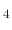) aging. It was found in  that thicknesses of the deposited SnOfilm decrease, when the time interval between its preparation and its use in the pyrolysis reaction increases. A suggestion is made to use either a freshly made solution or a completely aged solution during spray pyrolysis. During the presented topography simulations, it is assumed that the nozzle distance to the substrate, air pressure, and solution aging remains constant, while the spray is constantly applied. The nozzle's distance to the substrate is set to 20cm laterally and 10cm vertically, the air pressure to 1atm, and the solution is freshly prepared. In correspondence, the time and temperature dependences are investigated in the model.

Experimental data ,  suggest a linear dependence on spray time and a logarithmic dependence on wafer temperature for the growth rate of the deposited SnOlayer. A good agreement is given by the Arrhenius expression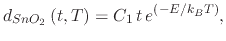(207)

where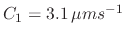, the thickness is given in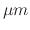,is the time in seconds, and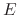is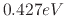. Figure 6.19 depicts the (a) experimental and (b) simulated topography of a deposited SnOfilm on a step structure after applying a PSD process for 45 seconds at 400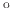C. The incoming flux is set to flow in the (1, -3) = (lateral, vertical) direction and a CVD-like process is simulated with a reaction order of 1 and a sticking probability of 0.2, as these values produced the best fit to the experimental data. The average direction of the initial flow is (1,0), but due to the effects of gravity in the vertical direction, the droplet flux experiences a downward acceleration. However, in the lateral motion, it only experiences the retardant Stokes force. Therefore, it is obvious that the direction of the flux will change from mainly horizontal to mainly vertical as it reaches the wafer.

L. Filipovic: Topography Simulation of Novel Processing Techniques